# Fractions Class 6 Extra Questions Maths Chapter 7

Fractions Class 6 Extra Questions Maths Chapter 7

### Fractions Class 6 Extra Questions Maths Chapter 7

Extra Questions for Class 6 Maths Chapter 7 Fractions

### Fractions Class 6 Extra Questions Very Short Answer Type

Question 1.
Represent the following fractions on number line.Solution: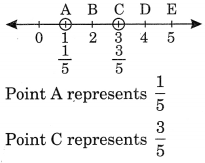Question 2.
Write the fractions showing the shaded portions: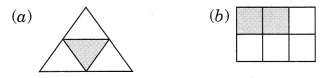Solution:

Question 3.
Colour the part according to the fraction given:Solution:Question 4.
Identify the proper and improper fractions:Solution:
Proper fractions are: 5/61/2 and 3/4
Improper fractions are: 7/211/5 and 6/5

Question 5.
What fraction of these circles have ‘x’ in them?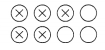Solution:
Fraction of the circles with ‘x’ in the given figure = 5/8.

Question 6.
Write all the natural numbers from 1 to 15. What fraction of them are prime numbers?
Solution:
Natural numbers from 1 to 15 are 1, 2, 3, 4, 5, 6, 7, 8, 9, 10, 11, 12, 13, 14 and 15 Prime numbers from 1 to 15 are 2, 3, 5, 7, 11, 13, i.e., 6 prime numbers.
∴ Fraction of prime numbers = 6/15

Question 7.
Identify the like fractions from the following: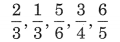Solution:
2/3 and 1/3 have the same denominator.
∴ 2/3 and 1/3 are the like fractions.

Question 8.
Identify the unlike fractions from the following:Solution:
2/5 , 2/7 and 1/6 have different denominators.
∴ 2/5 , 2/7 and 1/6 are unlike fractions.

Question 9.
Convert the following improper fractions into mixed fraction.Solution:Question 10.
Convert the following mixed fractions into improper fractions: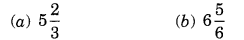Solution:### Fractions Class 6 Extra Questions Short Answer Type

Question 11.
Write the following fractions in ascending order:Solution:
Here, the numerators of all the fractions are same.Question 12.
Write any
(a) three proper and three improper fractions with denominator 7.
(b) two proper and two improper fractions with numerator 9.
Solution:
(a) Proper fractions with denominator 7 are: 2/7 , 3/7 and 5/7
Improper fractions with denominator 7 are: 9/7 , 11/7 and 13/6

(b) Proper fractions with numerator 9 are:
9/11 and 9/17
Improper fractions with numerator 9 are:
9/2 and

Question 13.
Compare the following fractions:Solution:Question 14.Solution:
LCM of 12, 16 and 24 is 48Question 15.Solution:Question 16.Solution:Question 17.
Insert > or < to make each of the following true.Solution: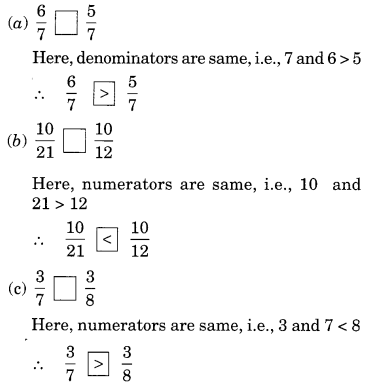### Fractions Class 6 Extra Questions Higher Order Thinking Skills (HOTS)

Question 18.
Find the difference between the greatest and the smallest fractions.Solution:Question 19.
Simran painted 2/3 of the wall space in her room. Her brother Rahul helped and painted 1/5 of the wall space. How much did they paint together? What part of the whole space is left unpainted?
Solution:
Space of the wall painted by Simran = 2/3
Space of the wall painted by Rahul = 1/5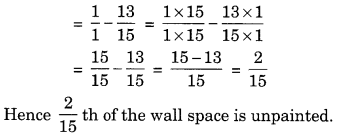## SabDekho

The Complete Educational Website